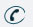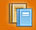781-366-0662

Subject

## Major Areas of Study

Number Sense and Operations : Students will understand numbers, ways of representing numbers, relationships among number, and number systems. They will understand the meanings of operations and how they relate to one another, compute fluently and make reasonable estimates.

Topics Covered : Integer and Rational Exponents, Exponential Growth and Decay, Define ‘e’, Laws of Logarithms, Solving Exponential Equations (including those using bases other than 10 or ‘e’)
In Level 1, topics studied also include: Understand the Polar Coordiante System and Graph Polar Equations, Convernt Between Rectangular and Polar Coordiantes, Write Complex Numbers in Polar Form, Derive and Apply Rules to Multiply Complex Numbers Using Their Polar Form, Derive and Apply MeMoivre’s Theorem for Computing Powers and Roots of Complex Numbers Using Their Polar Form.

Patterns, Relations, and Algebra : Students will understand patterns, relations, and functions. They will represent and analyze mathematical situations and structures using algebraic symbols, use mathematical models to represent and understand quantitative relationships, and analyze change in various contexts.

Topics Covered : Systems of Second Degree Equations, Graphs of Second Degree Equations, Definition of Conics as Locus of Points, Definition of Conics based on Eccentricity, Find Equations of Circles, Ellipses, Hyperbolas, and Parabolas and Create Corresponding Graphs, Values of Trigonometric Function for Special Angles, Approximate Values of Trigonometric Functions from Tables, Graphs of Trigonometric Functions, Equations of Lines With Direction Angle, Direction Angles of Conic Sections, Periodic Behavior,: Graphs, Equations, and problems, Simplify expressions with Multiple Trigonometric Functions: Using Reciprocals, Negatives, Cofunction and/or Pythagorean Relationships, or Algebraically and Graphically, Use the following to solve Equations and Create Graphs: Sin, Cos, Tan, Identify and Find Formulas and nth Terms of Arithmetic and Geometric Sequences, Define Sequences Recursively and Solve Problems Using Recursive Definitions, Apply Formulas for the Sum of the First n Terms of a Series, Find or Estimate Limits for Infinite Sequences, Identify Sequences With No Limits, Derive and Apply Formula for the Sum of an Infinite Geometric Series, Represent and Manipulate Series Using Sigma Notation, Find the Limit of a Function of the Quotient of Two Functions, Determine Whether a Function is Continuous, Sketch the Graphs of Rational Functions Using Limits
In Level 1, topics studied also include: Angle Sum, Angle Difference, Double Angle, and Half angle Relationship

Geometry : Students will analyze characteristics and properties of two-and three-dimensional geometric shapes and develop mathematical arguments about geometric relationships. They will specify locations and describe spatial relationships using coordinate geometry and other representational systems, analyze transformation and use symmetry to analyze mathematical situations. In addition, they will use visualization, special reasoning and geometric modeling to solve problems.

Topics Covered :Prove Theorems from Geometry Using Coordinates, Multi-Step Right Angle Triangle Problems, Area of Triangles with SAS, Derive and Apply Laws of Sines and Cosines to Triangles, Use Compass Headings to Solve Triangle Problems, Add and Subtract Vectors graphically and Algebraically, Multiply Vectors by Scalars Graphically and Algebraically
In Level 1, topics studied also include: Use Vector and Parametric Equations to Describe Motion in a Plane, Convert Parametric Equations to xy Equations, Describe the Dot Product of Vectors Graphically and Algebraically

Measurement :Students will understand measurable attributes of objects and the units, systems, and processes of measurement and apply appropriate techniques, tools, and formulas to determine measurements.

Topics Covered : Circle Definitions of Sin and Cos, Definitions of Tan, Cot, Cos, and Csc.

Data Analysis, Statistics, and Probability : Students will formulate questions that can be addressed with data and collect, organize and displace relevant data to answer them. They will select and use appropriate statistical methods t analyze data, develop and evaluate inferences and predictions that are based on data and understand and apply basic concepts of probability.

Topics Covered :Use Conditional Probabilities to Determine Independent Events. Use Binomial Theorem to Find Probability of Outcomes of a Binomial Experiment, Approximate a Non-Independent Probability with the Binomial Theorem, Solve Problems Involving Conditional Probability, Find the Expected Value where gains and Loses are Probabilistic, Use Expected Values to Determine if a Game is Fair
In Level 1, topics studied also include: Design Surveys and Apply Random Sampling Techniques to Avoid Bias in Data Collection, Apply Regression Results and Curve Fitting to Make Predictions From Data, Apply Uniform, Normal, and Binomial Distributions to the Solutions of Problems, Describe a Set of Frequency Distribution Data by Spread, Skewness, Symmetry, Number of Modes, or Other Characteristics.Subject Classes